Aishwarya Singh — Updated On April 20th, 2020

## How many boosting algorithms do you know?

#### Can you name at least two boosting algorithms in machine learning?

Boosting algorithms have been around for years and yet it’s only recently when they’ve become mainstream in the machine learning community. But why have these boosting algorithms become so popular?

One of the primary reasons for the rise in the adoption of boosting algorithms is machine learning competitions. Boosting algorithms grant superpowers to machine learning models to improve their prediction accuracy. A quick look through Kaggle competitions and DataHack hackathons is evidence enough – boosting algorithms are wildly popular!

Simply put, boosting algorithms often outperform simpler models like logistic regression and decision trees. In fact, most top finishers on our DataHack platform either use a boosting algorithm or a combination of multiple boosting algorithms.In this article, I will introduce you to four popular boosting algorithms that you can use in your next machine learning hackathon or project.

## 4 Boosting Algorithms in Machine Learning

2. Extreme Gradient Boosting Machine (XGBM)
3. LightGBM
4. CatBoost

## Quick Introduction to Boosting (What is Boosting?)

Picture this scenario:

You’ve built a linear regression model that gives you a decent 77% accuracy on the validation dataset. Next, you decide to expand your portfolio by building a k-Nearest Neighbour (KNN) model and a decision tree model on the same dataset. These models gave you an accuracy of 62% and 89% on the validation set respectively.

It’s obvious that all three models work in completely different ways. For instance, the linear regression model tries to capture linear relationships in the data while the decision tree model attempts to capture the non-linearity in the data.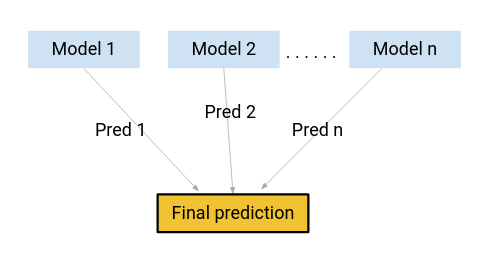How about, instead of using any one of these models for making the final predictions, we use a combination of all of these models?

I’m thinking of an average of the predictions from these models. By doing this, we would be able to capture more information from the data, right?

That’s primarily the idea behind ensemble learning. And where does boosting come in?

Boosting is one of the techniques that uses the concept of ensemble learning. A boosting algorithm combines multiple simple models (also known as weak learners or base estimators) to generate the final output.

We will look at some of the important boosting algorithms in this article.

## 1. Gradient Boosting Machine (GBM)

A Gradient Boosting Machine or GBM combines the predictions from multiple decision trees to generate the final predictions. Keep in mind that all the weak learners in a gradient boosting machine are decision trees.

But if we are using the same algorithm, then how is using a hundred decision trees better than using a single decision tree? How do different decision trees capture different signals/information from the data?

Here is the trick – the nodes in every decision tree take a different subset of features for selecting the best split. This means that the individual trees aren’t all the same and hence they are able to capture different signals from the data.

Additionally, each new tree takes into account the errors or mistakes made by the previous trees. So, every successive decision tree is built on the errors of the previous trees. This is how the trees in a gradient boosting machine algorithm are built sequentially.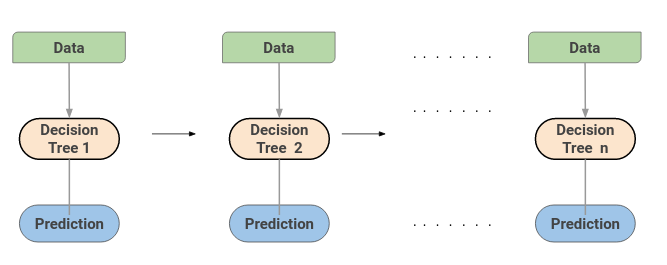Here is an article that explains the hyperparameter tuning process for the GBM algorithm:

## 2. Extreme Gradient Boosting Machine (XGBM)

Extreme Gradient Boosting or XGBoost is another popular boosting algorithm. In fact, XGBoost is simply an improvised version of the GBM algorithm! The working procedure of XGBoost is the same as GBM. The trees in XGBoost are built sequentially, trying to correct the errors of the previous trees.

Here is an article that intuitively explains the math behind XGBoost and also implements XGBoost in Python: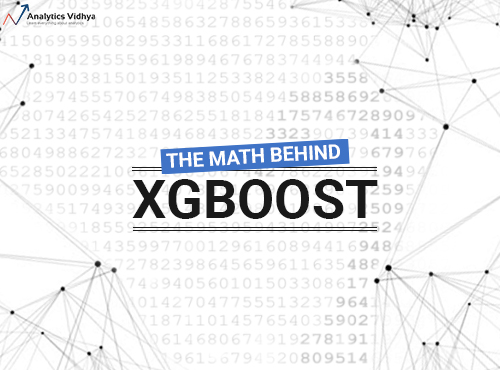But there are certain features that make XGBoost slightly better than GBM:

• One of the most important points is that XGBM implements parallel preprocessing (at the node level) which makes it faster than GBM
• XGBoost also includes a variety of regularization techniques that reduce overfitting and improve overall performance. You can select the regularization technique by setting the hyperparameters of the XGBoost algorithm

Learn about the different hyperparameters of XGBoost and how they play a role in the model training process here:

Additionally, if you are using the XGBM algorithm, you don’t have to worry about imputing missing values in your dataset. The XGBM model can handle the missing values on its own. During the training process, the model learns whether missing values should be in the right or left node.

## 3. LightGBM

The LightGBM boosting algorithm is becoming more popular by the day due to its speed and efficiency. LightGBM is able to handle huge amounts of data with ease. But keep in mind that this algorithm does not perform well with a small number of data points.

Let’s take a moment to understand why that’s the case.

The trees in LightGBM have a leaf-wise growth, rather than a level-wise growth. After the first split, the next split is done only on the leaf node that has a higher delta loss.

Consider the example I’ve illustrated in the below image: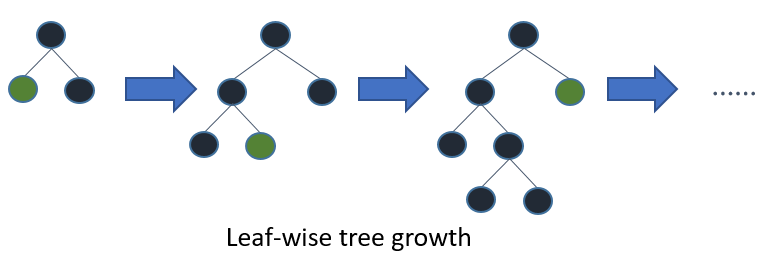After the first split, the left node had a higher loss and is selected for the next split. Now, we have three leaf nodes, and the middle leaf node had the highest loss. The leaf-wise split of the LightGBM algorithm enables it to work with large datasets.

In order to speed up the training process, LightGBM uses a histogram-based method for selecting the best split. For any continuous variable, instead of using the individual values, these are divided into bins or buckets. This makes the training process faster and lowers memory usage.

Here’s an excellent article that compares the LightGBM and XGBoost Algorithms:

## 4. CatBoost

As the name suggests, CatBoost is a boosting algorithm that can handle categorical variables in the data. Most machine learning algorithms cannot work with strings or categories in the data. Thus, converting categorical variables into numerical values is an essential preprocessing step.

CatBoost can internally handle categorical variables in the data. These variables are transformed to numerical ones using various statistics on combinations of features.

If you want to understand the math behind how these categories are converted into numbers, you can go through this article: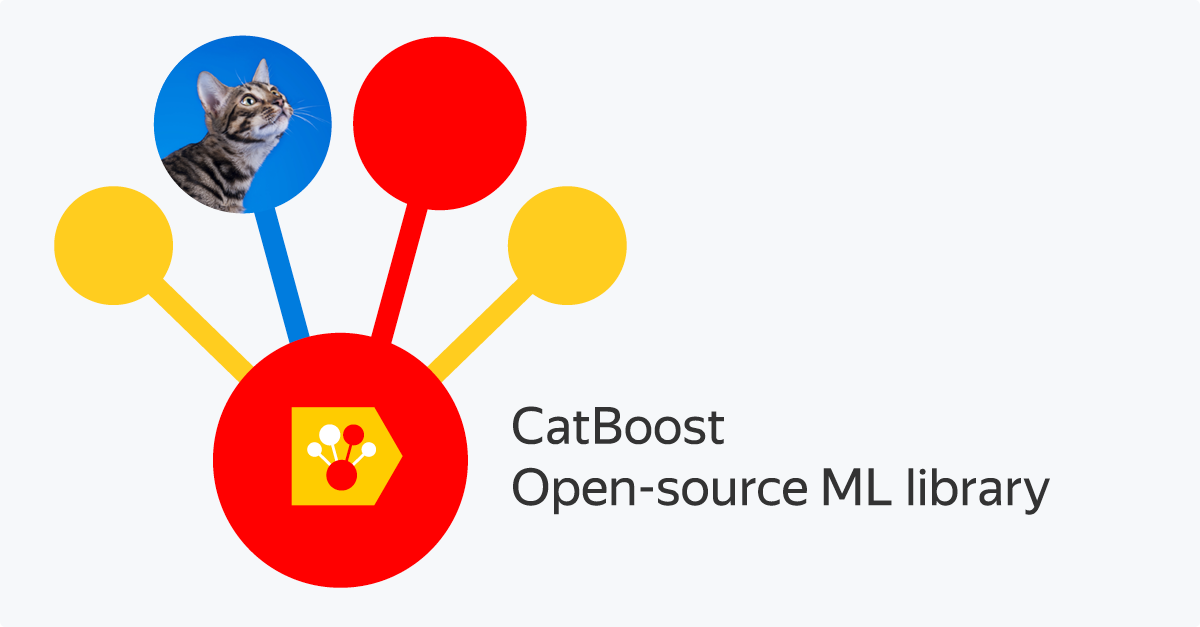Another reason why CatBoost is being widely used is that it works well with the default set of hyperparameters. Hence, as a user, we do not have to spend a lot of time tuning the hyperparameters.

Here is an article that implements CatBoost on a machine learning challenge:

## End Notes

In this article, we covered the basics of ensemble learning and looked at the 4 types of boosting algorithms. Interested in learning about other ensemble learning methods? You should check out the following article:

What other boosting algorithms have you worked with? Have you had any success with these boosting algorithms? Share your thoughts and experience with me in the comments section below.###### Aishwarya Singh

An avid reader and blogger who loves exploring the endless world of data science and artificial intelligence. Fascinated by the limitless applications of ML and AI; eager to learn and discover the depths of data science.

### Our Top Authors

•